# Module I(V) Curve

A complete module I(V) curve is computed under ambient conditions for the current time step. The “One-Diode” parameters are defined in the module file. The one-diode model uses an equivalent electrical circuit model and the governing diode equation to generate an explicit, continuous current vs. voltage function.

# One-Diode Model

Given the 1-diode model parameters for the module, this routine computes the module’s current-voltage characteristics. Figure 35 shows the equivalent circuit of a PV cell. The following algorithm includes the recombination current, Irec, a refinement in the model that can be set to zero if the parameters required to determine the current (μ, τeff, di, Vbi) are not provided in the module definition input file.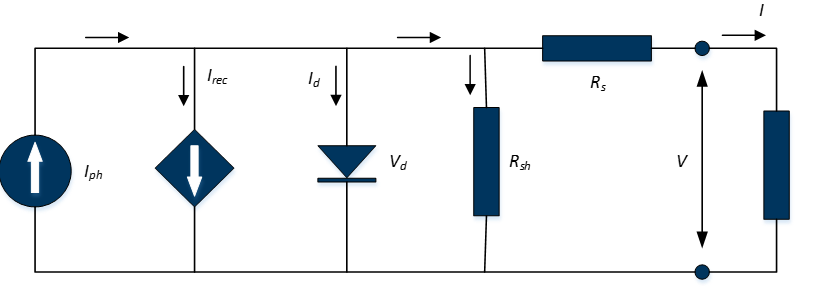## Inputs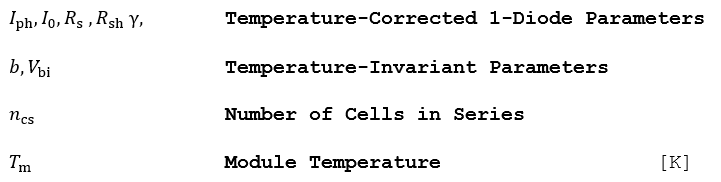## Outputs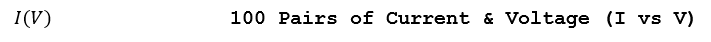## Algorithm

1.) Solve the following transcendental equation that relates the module’s output current i and output voltage υ to the photocurrent Iph, and the 1-diode parameters corrected for the temperature and irradiance level (I0, Rsh, Rs, γ).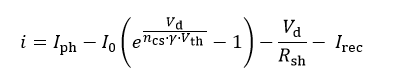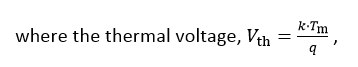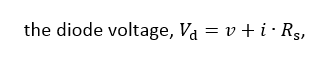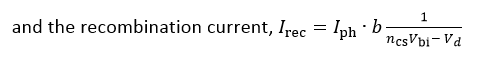The parameter b, given in the module parameter definition, combines the following terms: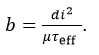Expanded, the transcendental function for the output current becomes: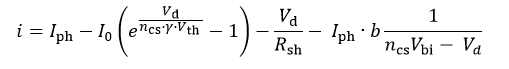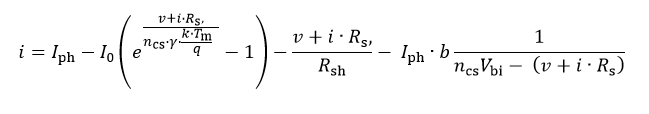2.) The current should be solved for diode voltages in the interval [0, νoc,g], where νoc,g is the guess for the open-circuit voltage, and can be found by setting the I to zero in the above equation (neglecting Irec):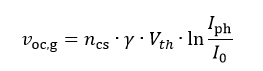3.) The limits of the I(V) curve in the first quadrant are defined by the open-circuit voltage and short-circuit current, which can be found by setting i and ν to 0, respectively.

4.) Find the maximum power point diode voltage using the Newton-Raphson method by iteratively solving the following equation until the difference between Vd,n+1 and νd,n is arbitrarily small.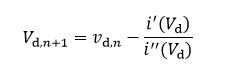5.) Find the maximum power point current by solving the 1-diode equation.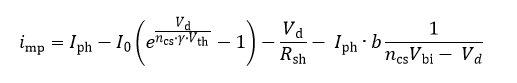6.) Find the maximum power point voltage at the output terminal of the equivalent circuit by subtracting the voltage drop across the series resistance.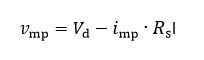## Reference

Mermoud, A., Conception et dimensionnement de systèmes photovoltaïques : Introduction des modules PV en couches minces dans le Logiciel PVsyst. Université de Genève, 2005.

# One-Diode Model Temperature Correction

Given the 1-diode model parameters defined for the module under STC conditions (25 °C, 1000 W/m2), compute the temperature and irradiance-corrected parameters at the actual module temperature Tm and the available solar energy GT,Eff. The default temperature correction of all 1-diode parameters is linear. If a non-linear temperature correction of the diode ideality factor is desired, the then a set of additional parameters (polynomial coefficients) is available to affect this correction.

This non-linear response is illustrated in Figure 36 for a family of I(V) curves with a module temperature range of 8 °C to 75 °C. This data was measured in the laboratory using the module temperature control system and a Spire long-pulse solar simulator.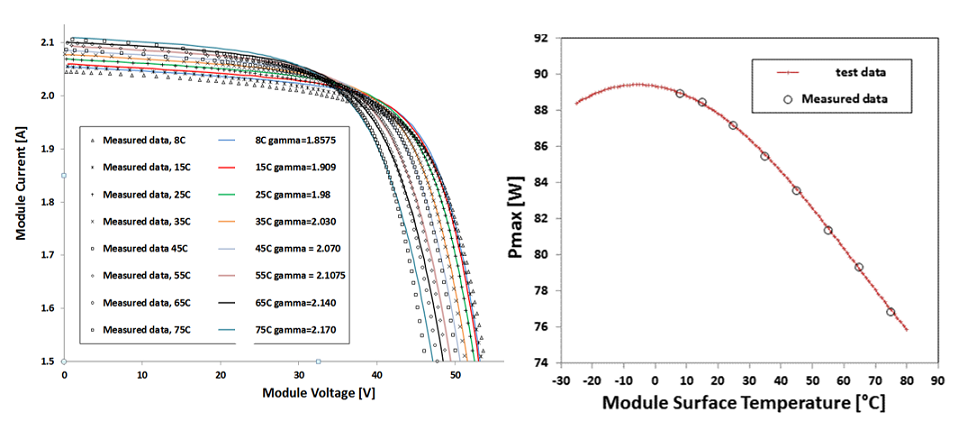## Inputs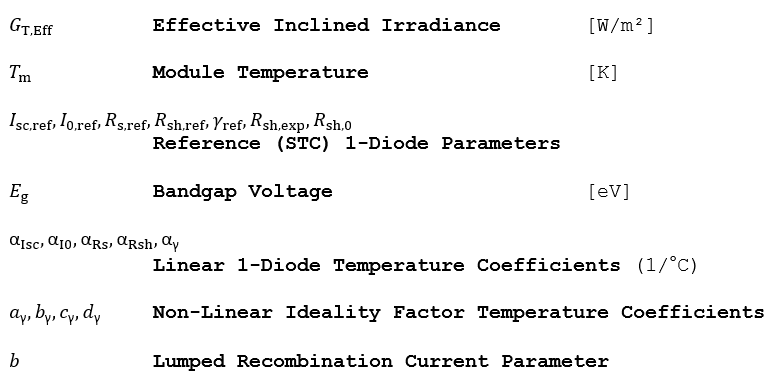## Outputs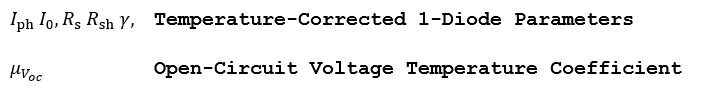## Algorithm

1.) Given the effective available insolation GT,Eff and module temperature Tm, find the corrected 1-diode shunt and series resistance.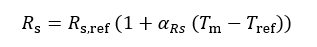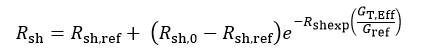2.) If the linear correction to the diode ideality factor is desired, or all non-linear coefficients ay,by,cy,dy = 0, then correct it for the module temperature as follows: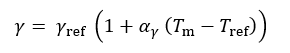3.) If the non-linear correction to the diode ideality factor is desired, then correct it for the module temperature as follows: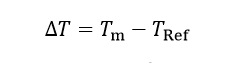4.) Correct the saturation current for temperature. Note that this correction includes the previously temperature-corrected diode ideality factor.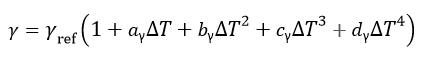*  In the above equation aγ is referring to the y1 non-linear temperature coefficient in PlantPredict and bγ is referring to y2, cγ is referring to y3 and dγ is referring to y45.) Find the short-circuit current under the ambient conditions.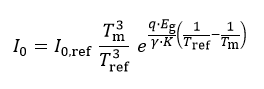6.) Even when a module is biased in short-circuit condition, a voltage drop occurs across Rs. As a result, the junction still experiences a voltage bias and there are currents flowing through both the recombination path and through Rsh when the terminal voltage of the device is zero, i.e. the recombination term does not go to zero in the short circuit condition. Therefore the photocurrent, Iph must be greater than Isc in order to supply parasitic currents as well as the external current which has the rated ISC,ref value. Find the actual Iph as follows: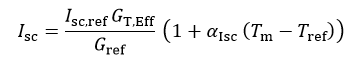The temperature dependence on voltage is also computed, but not used elsewhere in the simulation. Note that μΙsc ≅ αIph, given in 1/°C.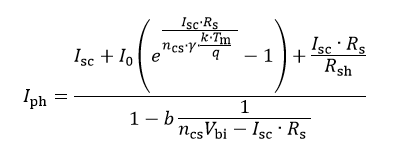## Reference

Schwieger, M., Michalksi, S., Non-Linearity of Temperature Coefficients, Equivalent Cell Temperature and Temperature Behaviour of Different PV-Module Technologies. TÜV Rheinland Energie und Umwelt, Cologne, Germany. Proceedings from the 28th EU PVSEC.